# MATH 575 Project .pdf

### File information

This PDF 1.5 document has been generated by TeX / pdfTeX-1.40.17, and has been sent on pdf-archive.com on 16/08/2017 at 23:16, from IP address 68.61.x.x. The current document download page has been viewed 431 times.
File size: 259.16 KB (16 pages).
Privacy: public file

### Document preview

MATH 575 - Project
Connor Davis, Youssef Katamish, Nicholas VanderLaan
August 16, 2017
In this final project we will explore the relation between a problem we discussed in class,
namely:
For what rational primes p is 7 a cube mod p?
and the Langlands program. When working on this project, you will at various points have to
take on faith something said below. Also, don’t be afraid to make educated guesses. (If you don’t
know the definition of something below, feel free to come by and ask me.)
1. First, recall our discussion in class, and write out a criterion for 7 to be a cube mod p in
terms of the splitting of p in the field Q(ω) using cubic reciprocity. (When p ≡ 1 mod 3, this
should depend on the congruence class of π (or π
¯ ) mod 7, where p = π¯
π is the decomposition
of p as a product of primary primes.) Use this to determine the set of primes less than 150
for which 7 is a cube mod p.
Proof. We consider two cases:
First the case where p ≡ 1(3): 7 splits in Z[ω], hereby referred to as D as the product of
(2 + 3ω)(−1 − 3ω) = λλ. Additionally, because p ≡ 1(3), p = ππ where π ∈ D, and π is the
conjugate of π. We can assume π is primary, else we can just split 7 differently in D. The
¯ =
conjugates of primary primes are primary =⇒ π is also primary. Then χπ (7) = χπ (λλ)
¯
χπ (λ)χπ (λ). By cubic reciprocity, we have that χλ (π)χλ (π). χπ (7) = 1 is true then when
either χλ (π) = χλ (π) = 1 or χλ (π) = ω, χλ (π) = ω 2 , or χλ (π) = ω 2 , χλ (π) = ω ./.
N (λ)−1

χλ (π) = π 3 (λ) = π 2 (λ). We can view equivalence classes mod λ as classes in Z/N (λ)Z
by the natural isomorphism. So
π
1
2
3
4
5
6

π 2 (λ)
1
ω
ω2
ω2
ω
1

π
1
2
3
4
5
6

π 2 (λ)
1
ω2
ω
ω
ω2
1

Thus we need to simultaneously satisfy the congruences given in ./. By the Chinese remainder theorem, we get the following:

1

factor
4+ω
3 + 6ω
1+ω
6 + 5ω
5 + 3ω
2 + 4ω

(π, λ)
(1, −1)
(−1, 1)
(2, −2)
(−2, 2)
(3, −3)
(−3, 3)

Second the case where p ≡ 2(3): Here we recall that the map
f : Z/pZ → Z/pZ
x 7→ x3
is an isomorphism, so 7 will always be congruent to a cube mod p.
Of the set of primes less than 150: {2, 3, 5, 7, 11, 13, 17, 19, 23, 29, 31, 37, 41, 43, 47}
∪{53, 59, 61, 67, 71, 73, 79, 83, 89, 97, 101, 103, 107, 109, 113, 127, 131, 137, 139, 149},
A := {2, 5, 11, 17, 23, 41, 47, 53, 59, 71, 83, 89, 101, 107, 113, 127, 139, 149} are congruent to
2 mod (3) =⇒ 7 is a cube mod p ∈ A. Of the remaining primes, 7 is congruent to a cube
only for {19, 73}.

2

2. Let q be a power of a prime p and let Fqn denote the finite field with q n elements. Show
that σ
¯ (x) = xq is a field automorphism of Fqn that acts as the identity on Fq . This is
called the Frobenius automorphism. The set of automorphisms of Fqn over Fq is naturally
a group, called the Galois group Gal(Fqn /Fq ). Show that this group has n elements and in
fact is cyclic of order n generated by σ
¯.
Proof. We will first show that σ
¯ (x) = xq is a field homomorphism. i.e. we need to show
that σ
¯ (0) = 0, σ
¯ (a + b) = σ
¯ (a) + σ
¯ (b), σ
¯ (ab) = σ
¯ (a)¯
σ (b).
The first equality is trivial.
Note that the characteristic of the field is p (since q is a power of p, i.e. q = pk for some
k ∈ Z+ ). Hence, we have that (a + b)p = ap + bp for all a, b ∈ Fqn . A simple inductive
x
x
x
argument shows that (a + b)p = ap + bp for all a, b ∈ Fqn and x ∈ Z+ . Thus, we have
k
k
k
n
that σ
¯ (a + b) = (a + b)q = (a + b)p = ap + bp = aq + bq = σ
¯ (a) + σ
¯ (b) for all a, b ∈ Fq
n

We have that σ
¯ (ab) = (ab)q = aq bq = σ
¯ (a)¯
σ (b) for all a, b ∈ Fq , since Fqn is a field and
hence multiplication is commutative.
We will now show that σ
¯ is a field automorphism. It suffices to show that σ
¯ is injective,
since σ
¯ is a field endomorphism to a finite field. Note that ker σ
¯ is an ideal in Fqn , meaning
that ker σ
¯ is trivial or Fqn . Since σ
¯ is a field endomorphism, ker σ
¯ = Fqn iff ker σ
¯ = 0. Since
σ
¯ (1) = 1, σ
¯ 6= 0 and hence ker σ
¯ = 0. Thus, σ
¯ is injective.
Hence, σ
¯ is a field automorphism.
We will now show that σ
¯ acts as the identity of Fq . Let x ∈ Fq . If x = 0, then σ
¯ (x) = 0q = 0.
q−1
q
×
= 1. Thus, this means that x = x and hence
If x 6= 0, then x ∈ Fq and hence x
σ
¯ (x) = x, as required.
Let Gal(Fqn /Fq ) represent the group of the set of all automorphisms of Fqn over Fq . We
will show that this group is a cyclic group of order n.
We will first prove a more general statement. We will prove that if L/K be a finite extension
of finite fields, where [L : K] = n, then | Gal(L/K)| ≤ n. Since L is a finite field, then L
is generated by an element α ∈ L. First note that α 6∈ K and hence α is the root of an
irreducible polynomial p(x) ∈ K[x] such that the degree of p is n. Thus, we have that any
automorphism must map α to a root of p. Since p can have at most n roots in L, we have
that there are at most n different automorphisms on L that fix K.
We will now show that σ
¯ k is distinct for all k ∈ {1, . . . , n − 1}. Recall that F×
q n is cyclic
2
n−1
of order q n − 1. Let α be the generator of Fqn . We have that: 1, αq αq , . . . , αq
are all
distinct elements. Hence, this means that σ
¯ (α), σ
¯ 2 (α), . . . , σ
¯ n−1 (α) are all distinct. Hence,
we have that σ
¯ k are distinct for all k ∈ {1, . . . , n − 1}.
Since [Fqn : Fq ] = n and since σ
¯ k is distinct for k ∈ {0, . . . , n−1}, we have that Gal(Fqn /Fq )
is a cyclic group of order n that is generated by σ
¯.

3

3. Consider the diagram of fields:
L = Q(ω, 71/3 )

F = Q(71/3 )

K = Q(ω)

Q
Then G = Gal(L/Q), which as above is defined to be the group of automorphisms of L/Q,
is a group of order 6 generated by σ and τ , where
σ(ω) = ω 2 ,
τ (ω) = ω,

σ(71/3 ) = 71/3
τ (71/3 ) = ω · 71/3 .

Show that G is isomorphic to S3 . Write down all the different conjugacy classes in G.
Proof. Recall from group theory that there are only two groups of order 6 up to isomorphism. The two groups are: Z/6Z and S3 . Note that Z/6Z is an abelian group and S3 is a
non-abelian group.
Hence, to show that G is isomorphic to S3 , it suffices to show that G is not abelian.
Let 71/3 ∈ L and consider σ ◦ τ and τ ◦ σ. We have that:
(σ ◦ τ ) (71/3 ) = σ(τ (71/3 )) = σ(ω · 71/3 ) = ω 2 ◦ 71/3
and

(τ ◦ σ) (71/3 ) = τ (σ(71/3 )) = τ (71/3 ) = ω · 71/3

Hence, this means that τ ◦ σ 6= σ ◦ τ and hence G is not abelian. Since G is a group of
order 6, G is isomorphic to S3 , as required.
Recall that two elements a, b ∈ G are conjugates if there exists g ∈ G such that g −1 ag =
b. From group theory, we know that conjugacy is an equivalence relation and hence the
partitions are called the conjugacy classes.
To compute the conjugacy classes of G, we will first write G in terms of the generators σ
and τ .
For notational purposes, from this point on, we will write στ to denote σ ◦ τ .
We have that στ 6= τ σ, hence those are two other distinct elements.
Note that σ 2 = Id, and τ 2 6= Id yet τ 3 = Id and hence G = {1, σ, τ, τ 2 , στ, τ σ}.
Before we proceed, we will compute a relation that will aid with the computation throughout
this problem. Note that στ 2 (ω) = σ(ω) = ω 2 and στ 2 (71/3 ) = σ(τ (ω · 71/3 ) = σ(ω 2 · 71/3 ) =
ω · 71/3 . Observe that τ σ(ω) = τ (ω 2 ) = ω 2 and τ σ(71/3 ) = τ (71/3 ) = ω · 71/3 . Hence, we
have that τ σ = στ 2 .
Let Cx denote the conjugacy class of x ∈ G.
Clearly, 1 is in a conjugacy class by itself (since g ∈ G implies that g −1 · 1 · g = g −1 g = 1).
Hence, we have that C1 = {1}

4

We will first compute the conjugacy class of σ. Note that τ −1 = τ 2 and hence τ 2 στ
τ (τ σ)τ = τ (στ 2 )τ = τ σ. Hence, this means that σ, τ σ ∈ Cσ . Similarly, τ στ 2 = (τ σ)τ 2
(στ 2 )τ 2 = στ and hence στ ∈ Cσ . Consider (τ σ)−1 = στ 2 , we have that στ 2 στ σ
(στ 2 )στ σ = (τ σ)στ σ = τ 2 σ = τ στ 2 = στ . Finally, (στ )−1 = τ 2 σ and hence τ 2 σσστ
τ 2 στ = τ σ. Thus, we have that Cσ = {σ, στ, τ σ}.

=
=
=
=

Finally, consider Cτ . We have that στ σ = σστ 2 = τ 2 and hence τ 2 ∈ Cτ . Thus, since the
conjugacy classes partition G, we have that C1 = {1}, Cσ = {σ, τ σ, στ } and Cτ = {τ, τ 2 }
are the conjugacy classes of G.

5

4. Let RL denote the ring of integers of L. Let p be a (non-zero) prime ideal in RL . Then
p ∩ Z = (p) is a prime ideal in Z and we get an extension of finite fields
RL /p = Fp

Z/pZ = Fp
Note that G acts naturally on RL and any element of G sends a prime ideal in RL to a
prime ideal. (Why?) Let Dp denote the subgroup of G given by
Dp = {θ ∈ G : θ(p) = p}.
Then there is a natural map
Dp → Gal(Fp /Fp ).
(Why?) FACT: This map is always surjective and if p 6= 3, 7, then this map is an isomorphism. In particular, in this case, we get a canonical element in G attached to p called
Frobp or the Frobenius at p, namely the one that corresponds to the (Frobenius) generator
of Gal(Fp /Fp ) defined in Problem 2.
Proof. We show that G acts as an automorphism on RL . Fix g ∈ G and α ∈ RL . Since
g ∈ G, we have that g(x + y) = g(x) + g(y) and that g fixes the elements of Q.
(a) g(α) ∈ RL
α is the root of a polynomial with integer coefficients:
αn + an−1 αn−1 + . . . + a0 = 0
To show that g(α) is also in RL , we see that
g(0) = g(αn + an−1 αn−1 . . . + a0 )
= g(αn ) + g(an−1 αn−1 + . . . + g(a0 )
= g(α)n + an−1 αn−1 + . . . + g(a0 )
Note that since 0, a0 ∈ Z, g(0) = 0, so we have that g(α) is the root to a polynomial
with integer coefficients, and is thus an algebraic integer.
(b) g is surjective over RL .
We want to show ∃β ∈ RL such that g(β) = α. We know that ∃γ ∈ L such that
g(γ) = α since g is an automorphism over L. Since g(γ) = α, we have that
g(γ)n + an−1 g(γ)n−1 + . . . + a1 g(γ) + a0 = 0
or, equivalently,

g(γ n + an−1 γ n−1 + . . . + a1 γ) + a0 = 0

6

We want to show that γ is itself an algebraic integer, meaning that γ is the root to a
polynomial with integer coefficients. We continue, noting that g −1 (a0 ) = a0
g(γ n + an−1 γ n−1 + . . . + a1 γ) = −a0
g −1 g(γ n + an−1 γ n−1 + . . . + a1 γ) = −g −1 (a0 )
γ n + an−1 γ n−1 + . . . + a1 γ = −a0
γ n + an−1 γ n−1 + . . . + a1 γ + a0 = 0
as desired.
This shows that g is an automorphism on RL , since injectivity comes for free: any injective
map is injective when restricted. Thus G acts naturally on RL insofar as it is the group of
automorphisms of RL .
Next, we show that for a prime ideal p in RL , g(p) is also a prime ideal. First we show that
g(p) is an ideal. We show it is closed under addition and multiplication.
(a) a, b ∈ g(p), a + b ∈ g(p)
Well, a, b ∈ g(p) =⇒ ∃c, d ∈ p such that g(c) = a, g(d) = b Then, p is an ideal so
c + d ∈ p, meaning g(c + d) = g(c) + g(d) = a + b ∈ g(p)
(b) a ∈ g(p), r ∈ RL
We proceed similarly. a ∈ g(p) =⇒ ∃c ∈ p such that g(c) = a. Since p is an ideal,
rc ∈ p. Thus g(rc) = rg(c) = ra ∈ g(p)
Thus g(p) is an ideal.
Next we show it is, in fact, a prime ideal. By definition, g(p) will be a a prime ideal if
for ab ∈ g(p), either a ∈ g(p) or b ∈ g(p). So, fix ab ∈ g(p) and assume that a 6∈ g(p).
Since ab ∈ g(p), ∃x ∈ p such that g(x) = ab. But since a 6∈ g(p), ∀y ∈ p, g(y) 6= a. We
want to show that there exists an z ∈ p such that g(z) = b. Well, p is a prime ideal and
g −1 (ab) = g −1 (a)g −1 (b) ∈ p. If g −1 (a) were in p, then we contradict that a 6∈ g(p). So
g −1 (b) ∈ p, which implies that b ∈ g(p).
Thus g(p) is a prime ideal.
Given that elements of G map prime ideals in RL to prime ideals, we can consider those
maps of G that map a particular prime ideal p to itself. Let
Dp = {θ ∈ G : θ(p) = p}
We show there exists a natural map φ : Dp → Gal(Fp /Fp ). Gal(Fp /Fp ) is the group of
automorphisms on Fp that fix Fp
Consider the map that sends θ([α]) 7→ [θ(α)]. First we show φ maps into Gal(Fp /Fp ). To do
this we show φ maps the identity to the identity, and that the resultant automorphisms are
in fact automorphisms. That is, they are additive, multiplicative, surjective, and injective.
Fix θ ∈ Dp .
(a) identity to identity:
φ(θ()) = [θ(1)]
as θ is an automorphism

= 
7

[θ(α) + θ(β)] = φ(θ([α]) + θ[β])
= φ(θ([α])) + φ(θ([β]))
= [θ(α)] + [θ(β)]
(c) Multiplicative:
[θ(r · α)] = φ(θ([r · α])
= r · φ(θ[α])
= r · [θ(α)]
(d) Injective: Assume that [θ(α)] = [θ(β)]. We have
0 = [θ(α)] − [θ(β)]

hypothesis

= [θ(α) − θ(β)]

= [θ(α − β)]
So, α = β. Thus [θ(x)] is injective.

(e) Surjective: Follows from injectivity and the fact that Gal(Fp /Fp ) is a finite set.
And note that these automorphisms are well defined since θ ∈ Dp fix p and as θ ∈ G, they
send algebraic integers to algebraic integers.
Next, we show that φ is a homomorphism. We see that φ takes the identity automorphism
to the identity automorphism in Gal(Fp /Fp : Fix some α ∈ RL , so that [α] is a conjugacy
class in Fp . We have
φ(e[α]) = [e(α)]
= [α]
Recall that G = Gal(L/Q). If θ ∈ Dp ⊂ G, then θ(p) = p. Hence, θ maps a specific prime
ideal to itself.
The natural map φ : Dp → Gal(Fp /Fp ) can be given by φ(θ) = θ|Fp .
Note that θ|Fp is constant over Fp , as Fp ⊂ Q and θ ∈ Gal(L/Q).
To show that φ is a homomorphism, we will show that φ respects group operations:
φ(θ1 θ2 ) =
= (θ1 θ2 )|Fp
= (θ1 )|Fp + (θ2 )|Fp
= φ(θ1 )φ(θ2 )
as required.

8

5. Given any prime p in Z, there exists a prime p in RL such that p ∩ Z = (p). Then one gets
an element Frobp above which may very well depend on the choice of p above (p). However,
the conjugacy class of Frobp in G only depends on p !! This gives a conjugacy class in
G called Frobp . How does Frobp depend on the congruence class of p mod 3? (Make an
educated guess based on your knowledge of the cubic residue symbol and justify it as much
as possible.)
We have
√ two cases, p ≡ 1(3), p ≡ 2(3). We deal with the former first. Fix p ≡ 1(3). Set
α = 3 7. Let p be a prime ideal in RL such that p ∩ Z = (p). Recall that the Frobenius
automorphism σ(x) = xp , so σ(α) = αp . We compute
σ(ω) = ω p ≡ ω
But this is ambiguous, since it does not tell us if σ corresponds to a class that is the identity,
or that of a rotation. Thus we proceed further. Since p ≡ 1(3), we know that when p ≡ 1(3),
p splits into π and π over the Eisenstein integers, meaning π, π live in Q[ω]. Without loss of
generality, assume that π is primary. Also, we recall our definition of the cubic reciprocity
symbol. We have that
 
N y−1
x
=x 3
mod y
y
Thus, since N π = p,

 
p−1
7
=7 3
π

mod π = αp−1

mod π

Multiplying by α on both sides, we have that
 
7
α = αp mod p = σ(α)
π
where we are modulo p not π since α ∈ RL \RK . Then, the most right hand side is the Frobp .
We see that the LHS has two terms, α and the cubic reciprocity symbol which evaluates
to either {1, ω, ω 2 }, each of which is in its own conjugacy class. Then since multiplying by
α does not change the conjugacy class of either 1, ω, ω 2 , and the LHS depends only on p
(based on p’s splitting into π), then

 
7

=1
α, if

π

 

7
σ(α) = αω, if

π

 

7

= ω2
αω 2 , if =
π
Recalling that σ(ω) = ω, if we are in the first case that σ(α) = α, then we have that
Frobp is the identity, and is therefore in such a conjugacy class. In the other two cases, it
corresponds to a rotation element, and is therefore in such a conjugacy class.
Next, we consider p ≡ 2(3) This is very simple as σ(ω) ≡ ω p = ω 2 . Thus the Frobp is a
reflection conjugacy class.

9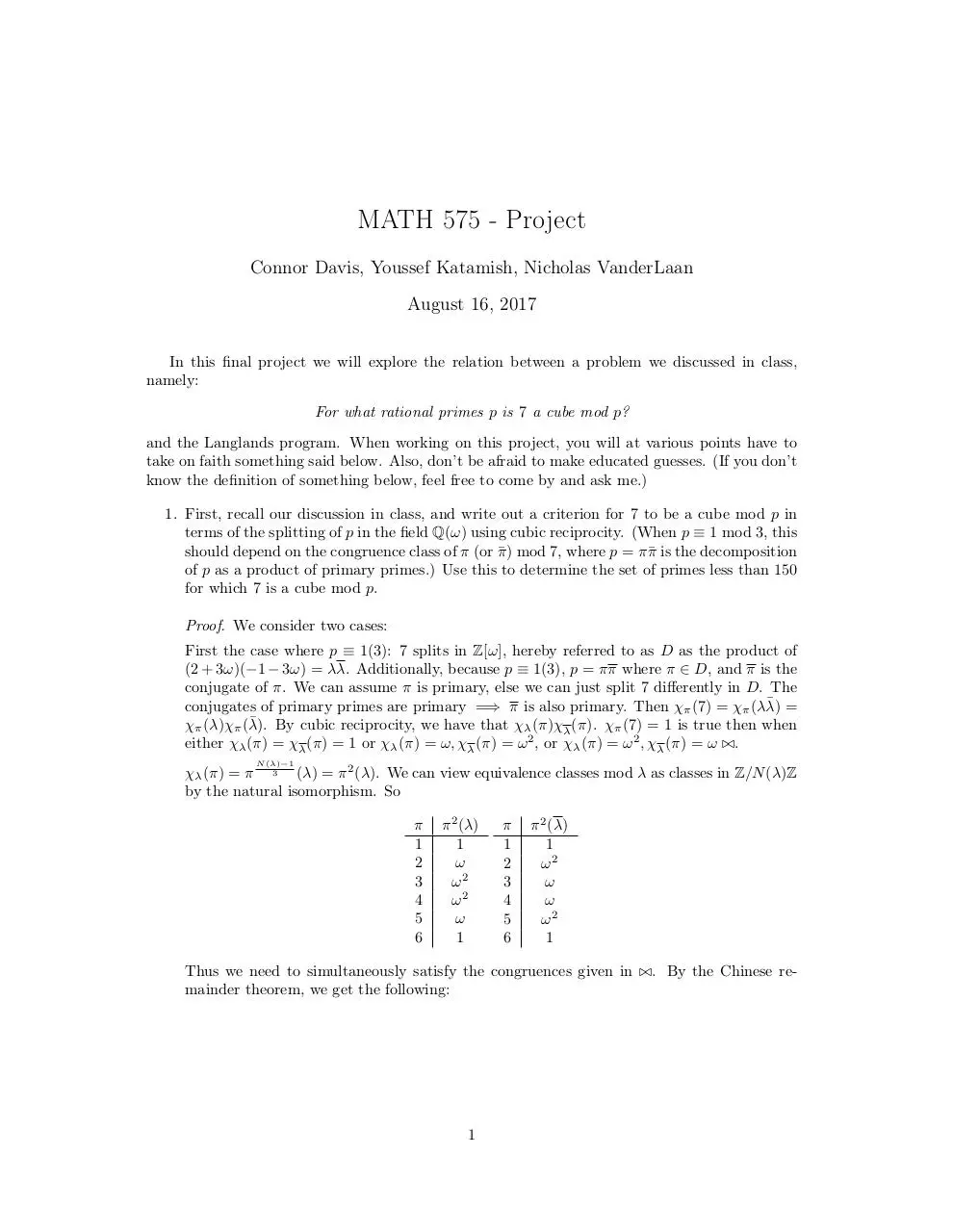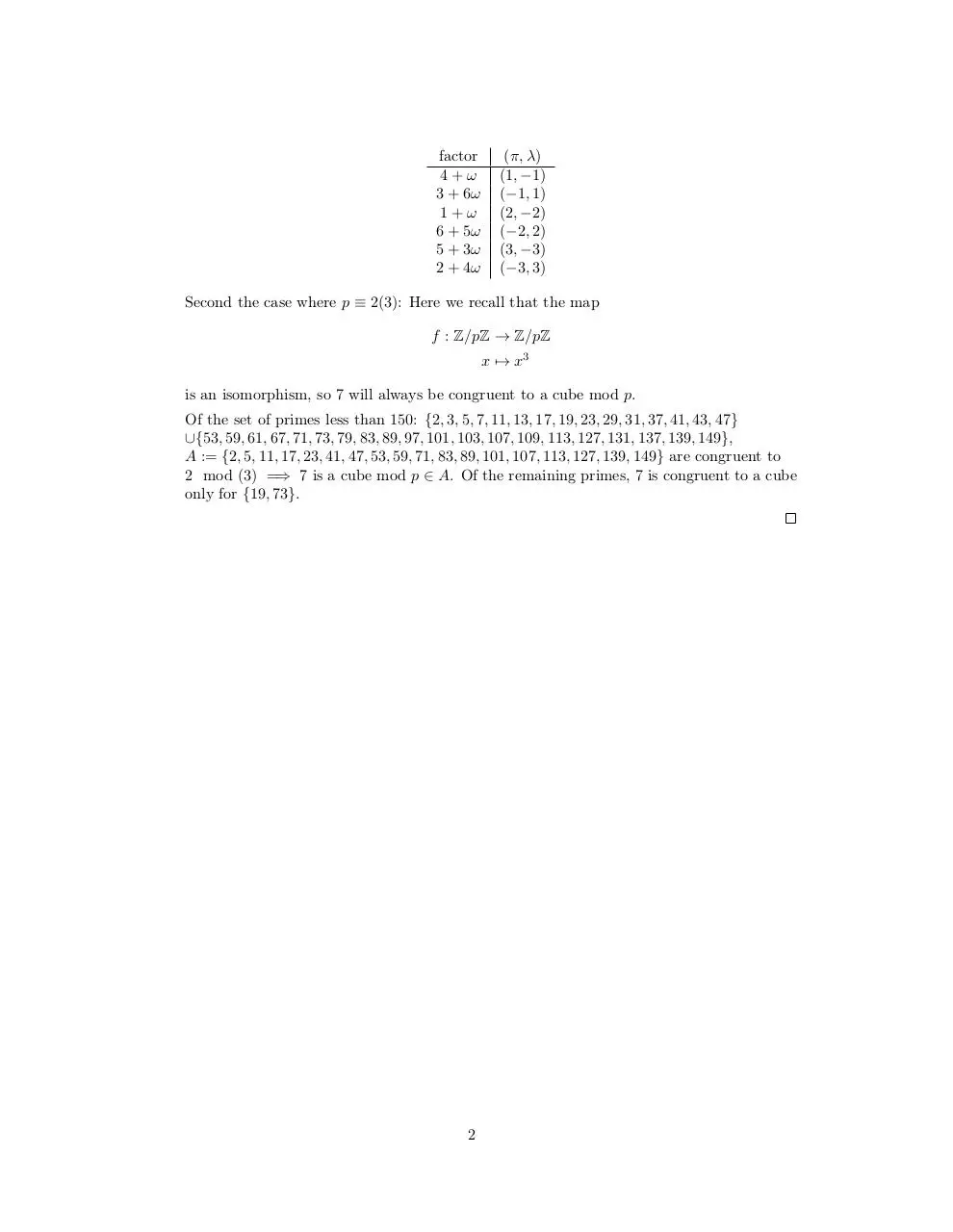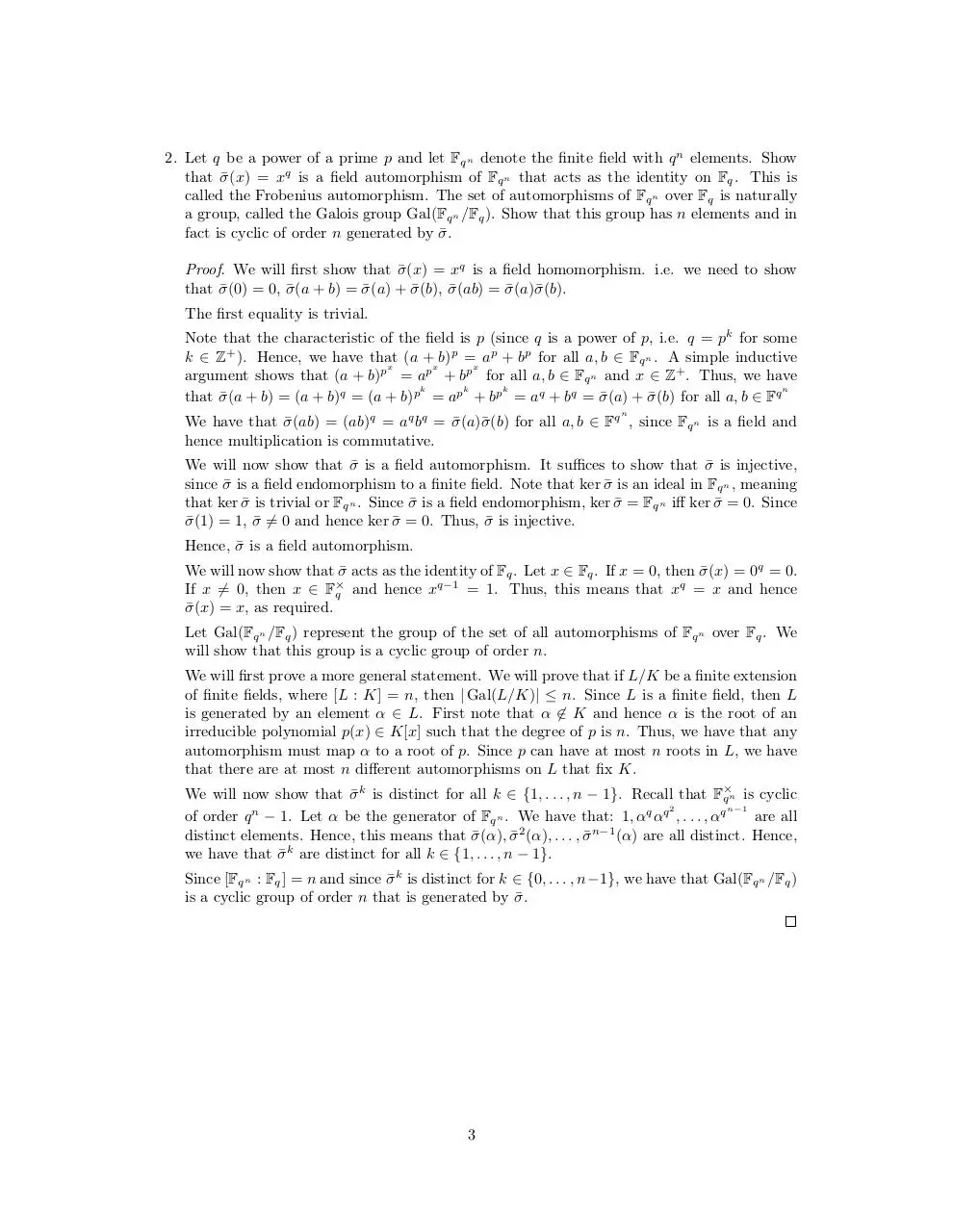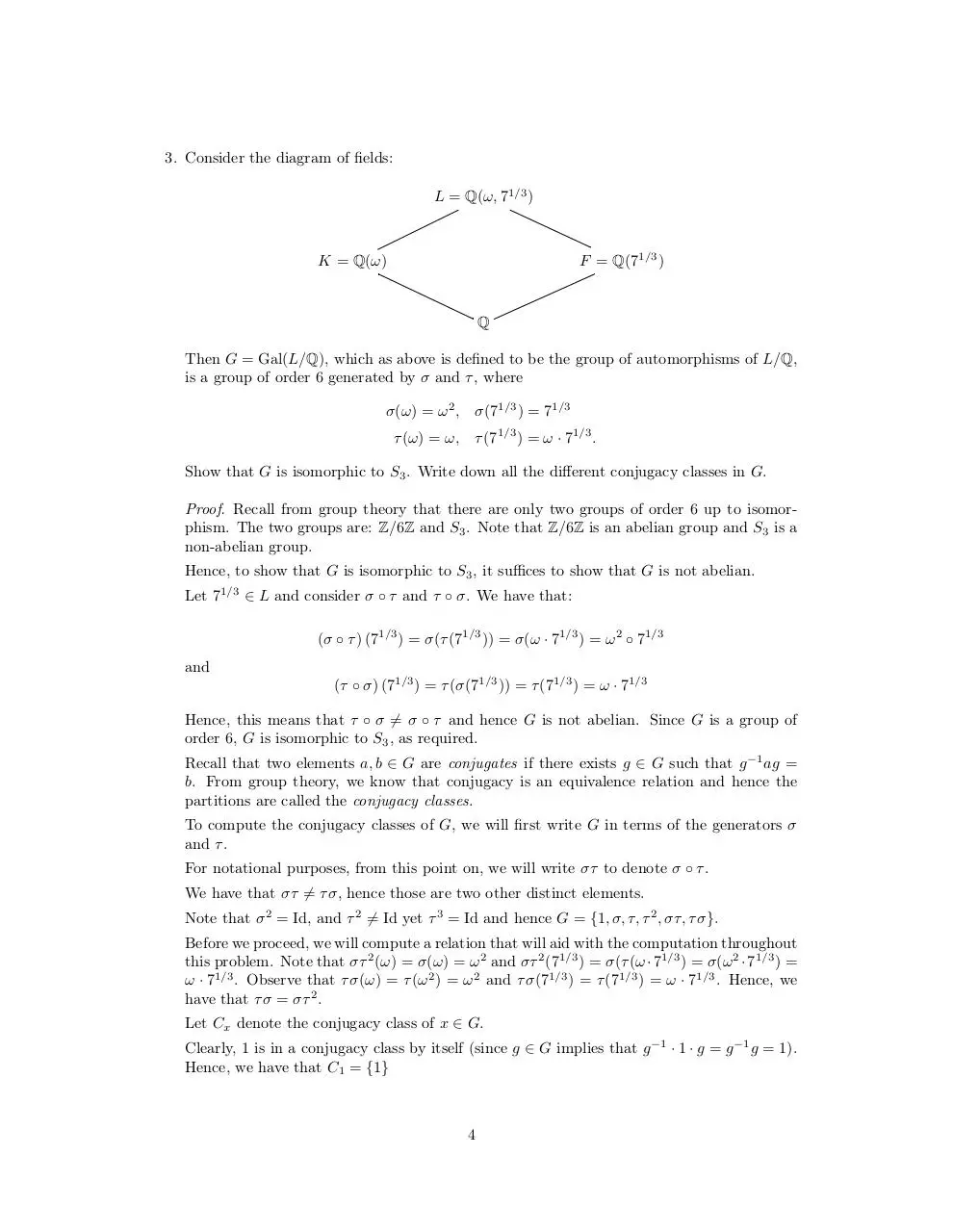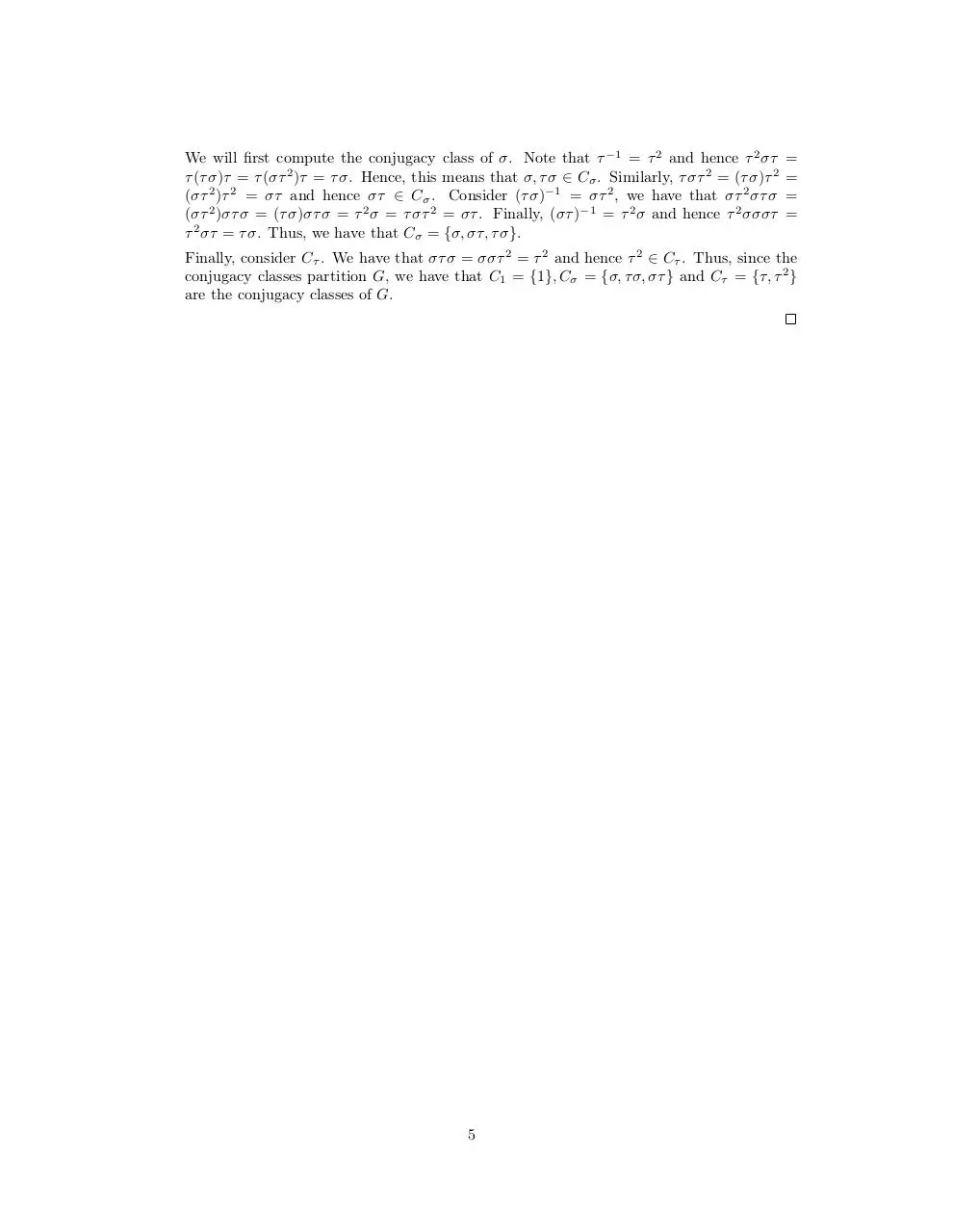MATH_575___Project.pdf (PDF, 259.16 KB)

### Share on social networks

#### HTML Code

Copy the following HTML code to share your document on a Website or Blog